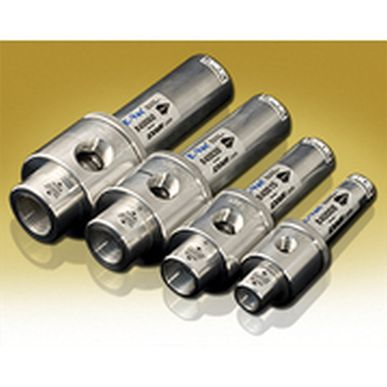# EXAIR E-Vacs真空產生器抽真空時間 Vacuum Evacuation Time for E-Vacs

BLOG EXAIR E-Vacs真空產生器抽真空時間 Vacuum Evacuation Time for E-Vacst =撤離時間（min）
V =封閉體積（ft ^ 3）
p0 =大氣壓（“Hg”）
p1 =最終真空壓力（“Hg”）
q =真空泵流量（SCFM）

V = 56.1英尺3（以上）
p0 = 29.92”Hg（絕對起始）
p1 = 8.92”Hg（絕對目標）
q = 70 SCFM（80 psig）

t = V * ln（p0 / p1）/ q
t = 56.1 ft ^ 3 * ln（29.92”Hg / 8.92”Hg）/ 70 SCFM
t = 0.97分鐘